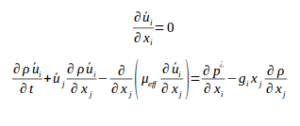+34 942 20 16 16 ihfoam@ihcantabria.com
Select Page

# Fluid Flow

## Introduction

IHFOAM solves the three-dimensional Reynolds Averaged Navier-Stokes (RANS) equations for two incompressible phases using a finite volume discretization and the volume of fluid (VOF) method. In VOF, each phase is described by a fraction α occupied by the volume of fluid in the cell. This technique allows very complex free surface configurations to be represented easily and involves no mesh motion. It also supports several turbulence models (e.g. κ−ε , κ−ω SST, LES).

## Governing equations

The aforementioned RANS equations include continuity and mass conservation equations, which are presented below. The assumption of incompressible fluids has been used, which is applicable for most coastal engineering practical problemsAlong with these governing mathematical expressions, which describe the motion of fluid flow, an additional one must also be taken into account to describe the movement of the phases.

## Field calculation

IHFOAM can obtain the following fundamental variables in any cell of the mesh:

• VOF function.
• Velocity field.
• Pressure field
• Turbulent magnitudes.

Several derived magnitudes can be obtained out of the box, either directly or using the post-processing GUI.  Those magnitudes are related with the functionality and stability of coastal structures:

• Functionality:
• Free surface elevation along the whole domain
• Free surface gauges and spectra
• Run-up on a slope (breakwater or beach)
• Overtopping analysis: mean overtopping discharge, instantaneous overtopping discharge, instantaneous volume discharge, mean and instantaneous velocity during overtopping events and thickness of the overtopping mass of water.
• Transmitted energy leeward the structures by overtopping and throughout the porous media
• Stability:
• Time evolution of the pressure law around monolithic structures (crown-walls and vertical breakwaters)
• Time evolution of the forces (horizontal and uplift forces) and moments
• Time evolution of the safety factor coefficient

Additional magnitudes related with surf zone hydrodynamics can be also calculated:

• Gravity and infragravity wave evolution
• Wave breaking
• Undertow
• Bottom shear stress